Get instant live expert help with Excel or Google Sheets“My Excelchat expert helped me in less than 20 minutes, saving me what would have been 5 hours of work!”

#### Post your problem and you’ll get expert help in seconds.

Your message must be at least 40 characters
Our professional experts are available now. Your privacy is guaranteed.

# How to Count Duplicate Values in Excel

Working with large data sets often requires you to count duplicates in Excel. You can count duplicate values using the COUNTIF function. In this tutorial, you will learn how to count duplicates using this function.

## How to Count Duplicates in Excel

You can count duplicates using the COUNTIF formula in Excel. There are a few approaches counting duplicates. You might want to include or exclude the first instance when counting duplicates. In the next sections, you will see some examples related to counting duplicates.

### How to Count Duplicate Instances Including the First Occurrence

The following example includes the data on student grades. The data contains the student name, age, and grades. Column D has the unique grades for which you are going to count the duplicates.

To find the count of duplicate grades including the first occurrence:

• Go to cell F2.
• Assign the formula `=COUNTIF(\$C\$2:\$C\$8,E2)`.
• Press Enter.
• Drag the formula from F2 to F4.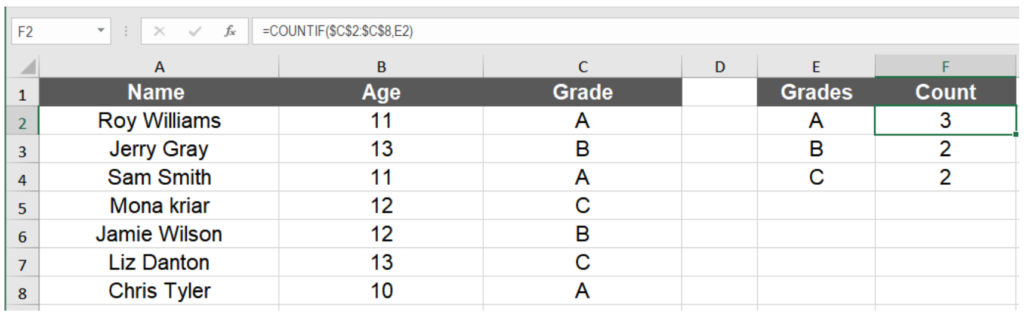Now you have the count for duplicate grades in column E.

### How to Count Duplicate Instances excluding the First Occurrence

Often you might need to calculate the number of duplicates in your data without the first occurrence. You can count the number of duplicates excluding the first entry in the same way as the previous example. To count the duplicate examples from the last example without the first occurrence:

• Select cell F2.
• Assign the formula `=COUNTIF(\$C\$2:\$C\$8,E2)-1`.
• Press Enter to apply the formula.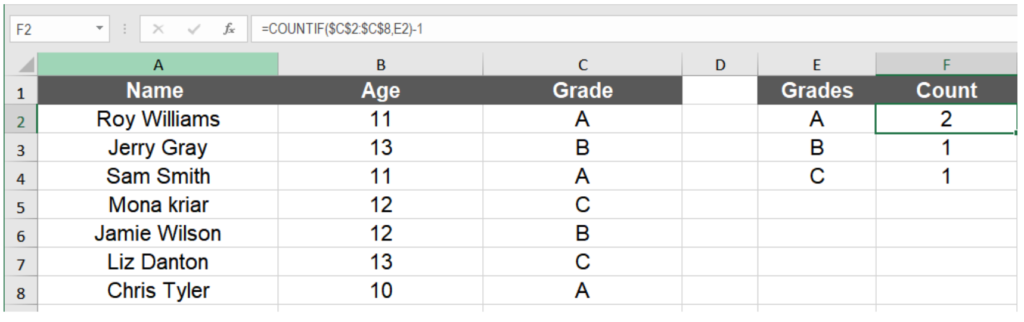This will show the count of duplicate values without the first instance in column E.

### Count Case-Sensitive Duplicates in Excel

The COUNTIF function in Excel is case-insensitive. You won’t get the actual count if you use it to count a case-sensitive duplicate. But you can use a combination of the SUM and EXACT function to get a case-sensitive count for duplicate instances. To find a case-sensitive count for duplicate values:

• Go to cell F2.
• Assign the formula `=SUM(--EXACT(\$C\$2:\$C\$8,E2))`.
• Press Ctrl + Shift + Enter to apply the formula as an array formula.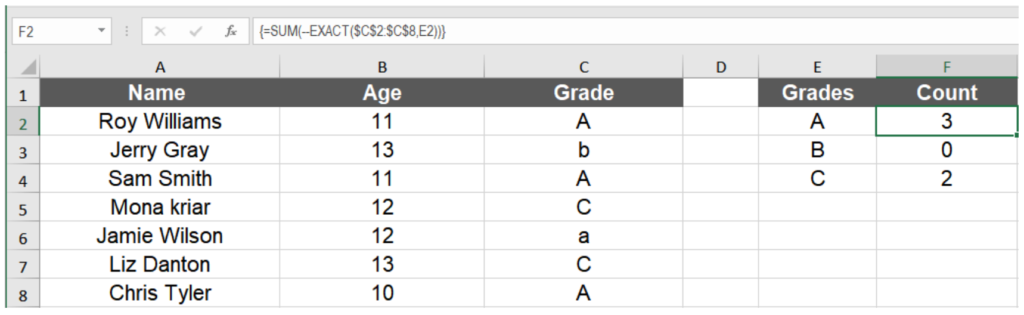The EXACT formula performs a case-sensitive compare for the values in column D with the grades in C2 to C8. This results in an array of logical values TRUE and FALSE. The unary operator (–) transforms the values to an array of 0 and 1’s. The SUM function then adds up these entries to find the count for the duplicate values.

### Counting Duplicate Rows in Excel

You can count duplicate rows that have the same values in every cell. This comes in very handy if you have a large dataset and want to identify duplicate rows for future modification. The COUNTIFS function lets you count based on multiple conditions. You will use the COUNTIFS function to count duplicate rows.

In the following example, you will use the student information. The data has the columns for the student names, age, and genders. Column E has all the unique names for which you will count the duplicate rows.

• Select the cell F2 by clicking on it.
• Assign the formula `=COUNTIFS(\$A\$2:\$A\$8,A2,\$B\$2:\$B\$8,B2,\$C\$2:\$C\$8,C2)` to F2.
• Press Enter.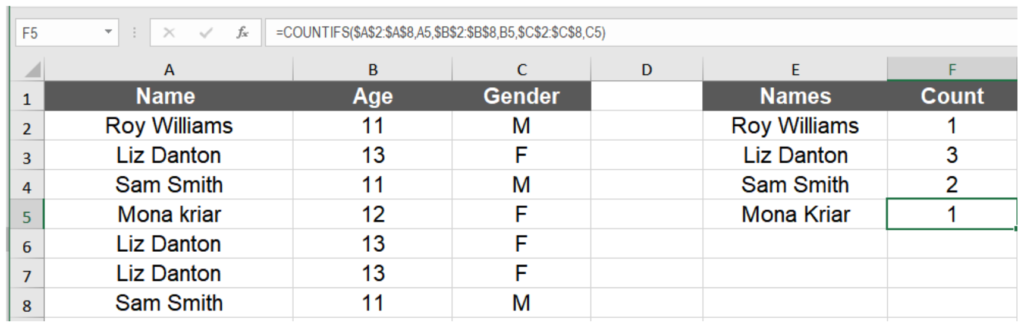Drag the formula to the cells below with your mouse.

### How to Count the Total Number of Duplicates in a Column

You can count the total of duplicates in a column in two steps. First, you need to identify all the duplicates in a column. Then you need to count these values. The next example includes different country names containing duplicates. To find the total number of duplicates without the first occurrence:

• Go to cell B2 by clicking on it.
• Assign the formula `=IF(COUNTIF(\$A\$2:A2,A2)>1,"Yes","") `to cell B2.
• Press Enter. This will show the value Yes if the entry in A2 is a repeated entry.
• Drag down the formula from B2 to B8.
• Select cell B9.
• Assign the formula` =COUNTIF(B2:B8,"Yes") `to cell B9.
• Hit Enter.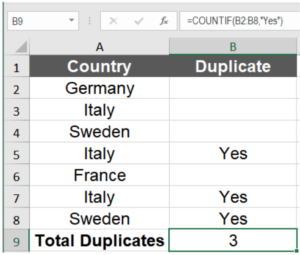This will show the total count of duplicate values in the column A without the first occurrence.

To count the duplicate values including the first occurrence:

• Select cell D4.
• Assign the formula `=ROWS(\$A\$2:\$A\$8)-SUM(IF(COUNTIF(\$A\$2:\$A\$8,\$A\$2:\$A\$8) =1,1,0))` to row B2.
• Press Ctrl + Shift + Enter.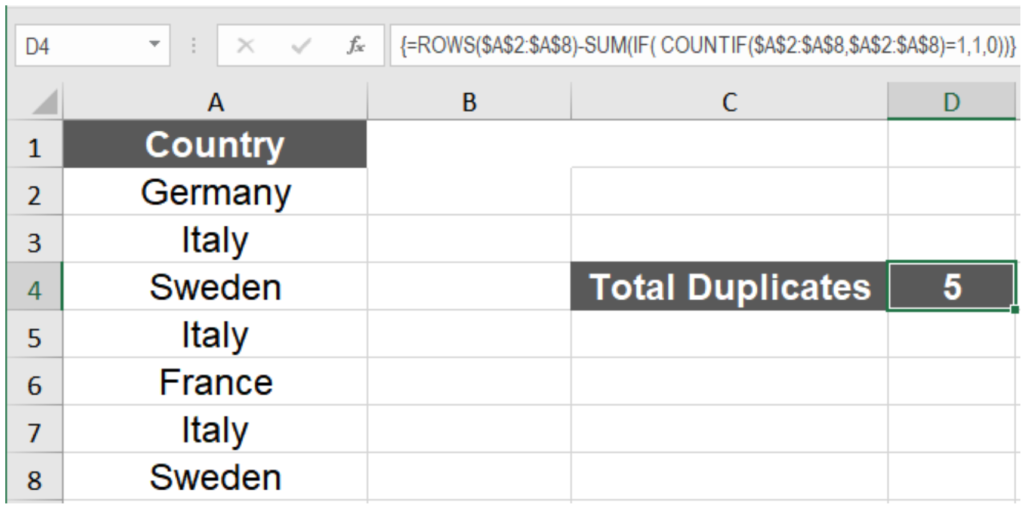Here we used the ROWS, SUM, and IF functions along with the COUNTIF function and apply it as an array function. This formula can be broken down into two parts. In the first step, the ROWS function counts the number of rows between A2:A8, which is 7. In the second part of the formula, the COUNTIF function is used to count the total matches in the range A2 to A8 with itself, and we nest all of this inside an IF function.

The condition used here is to return a 1 if it is a match and a 0 otherwise. The resulting 1’s are then summed, and the sum results in 2, which is the number of distinct matches between A2 to A8 with itself. The difference between these two steps returns 5, the total count of duplicates including the first instances.

Counting duplicates in Excel is a very handy trick when working with large datasets. The COUNTIF and COUNTIFS functions come in handy to count duplicate values in Excel and gain useful insights about the data.

Still need some help with Excel formatting or have other questions about Excel? Connect with a live Excel expert here for some 1 on 1 help. Your first session is always free.

### Did this post not answer your question? Get a solution from connecting with the expert.Another blog reader asked this question today on Excelchat:
Solution exampleshow can i turn my sumifs into a countifs because my sumifs works great and i also want to count the thing im summing
Solved by O. C. in 40 minsI am trying to have a tab change color when the Sum of a row = 0. I am using code on the tab but it appears to be confused because the value of the given cell is not 0 it is "=SUM(G4:G17)"
Solved by S. F. in 41 minsCould you help me by explaining the IF function?
Solved by G. L. in 24 minsI have a question about Conditional Formatting. =IF('Sheet1'!A1="X",IF('Sheet1'!B1="Y", TRUE, FALSE),FALSE) the set the cell background to red. I would like to make it so that if Sheet1'!B1="Z" I set the back to blue. How can I achieve this.
Solved by B. F. in 39 minsI need to find out if the 2018 yearly sales goals were met if the yearly sales were \$25,000 or more using an IF logical function and to set the formula to return a value of YES if met, and NO if not
Solved by A. A. in 18 mins## Subscribe to Excelchat.coAnother blog reader asked this question today on Excelchat: